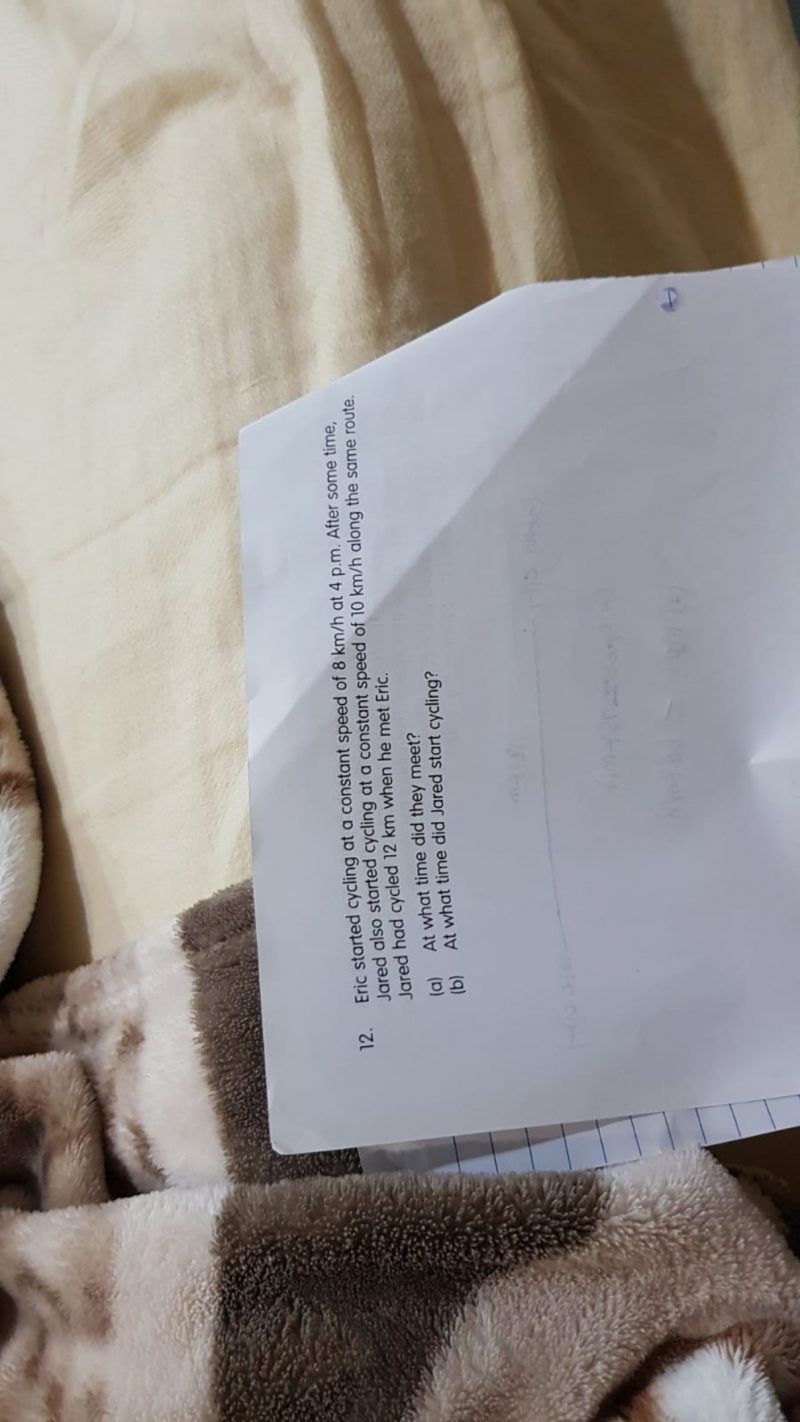# QuestionHi,

Another question also needs help .

Thanks

(a)
Jared cycled 12 km and met Eric.
Jared’s speed was 12 km/h.
Therefore, the time he needed to cycle 12 km:
Time = 12/10 = 1.2 hr

But after 1.2 hr, Eric only cycle a distance of:
Distance = 1.2 x 8 = 9.6 km

So,
12 km – 9.6 km = 2.4 km
The time Eric needed to cycle 2.4 km:
Time = 2.4/8 = 0.3 hr

So the time where both of them met will be:
1.2 hr + 0.3 hr = 1.5 hr
4 pm + 1 hr 30 mins = 5.30 pm

(b)
0.3 hr x 60 mins = 18 mins
4 pm + 18 mins = 4.18 pm